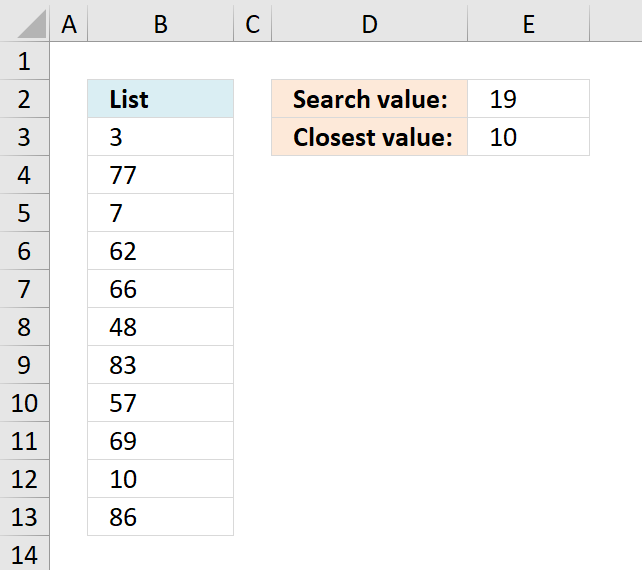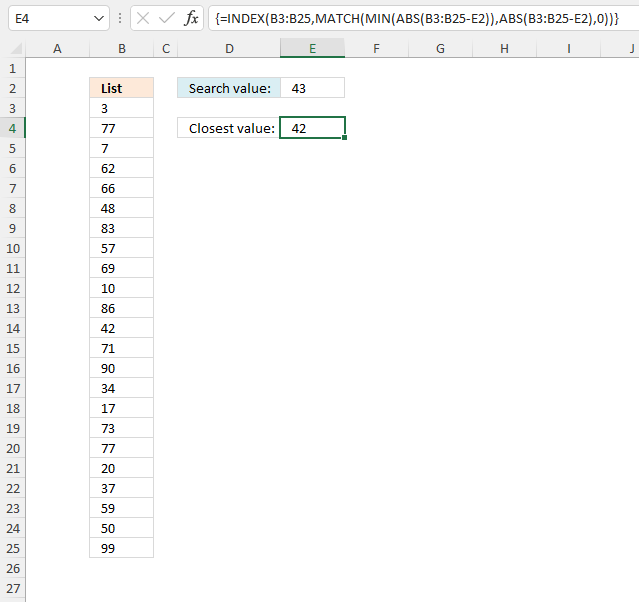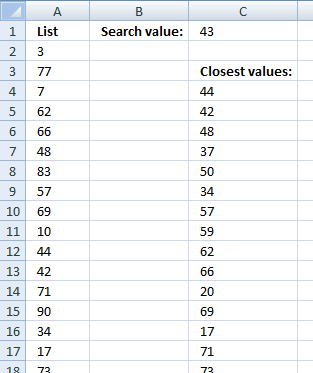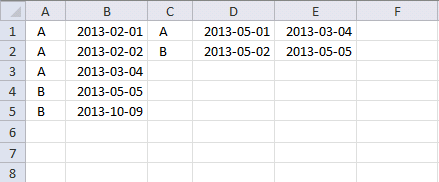Author: Oscar Cronquist Article last updated on June 28, 2022This article demonstrates formulas that extract the nearest number in a cell range to a condition. The image above shows a formula in cell E3 that calculates the nearest number in cell range B3:B13 to the number specified in cell E2.

## 1. Find the closest valueThe image above demonstrates a formula in cell E4 that extracts the closest number to the given number in cell E2.

Array formula in cell E4:

=INDEX(B3:B25, MATCH(MIN(ABS(B3:B25-E2)), ABS(B3:B25-E2), 0))

### 1.1 How to create an array formula

1. Copy (Ctrl + c) and paste (Ctrl + v) array formula into formula bar.
2. Press and hold Ctrl + Shift.
3. Press Enter once
4. Release all keys

### 1.2 Explaining array formula

#### Step 1 - Subtract numbers with search number

The minus sign lets you subtract numbers in an Excel formula.

B3:B25-E2

becomes

{3; 77; 7; 62; 66; 48; 83; 57; 69; 10; 86; 42; 71; 90; 34; 17; 73; 77; 20; 37; 59; 50; 99} - 43

and returns

{-40; 34; -36; 19; 23; 5; 40; 14; 26; -33; 43; -1; 28; 47; -9; -26; 30; 34; -23; -6; 16; 7; 56}

#### Step 2 - Remove negative sign

The ABS function converts negative numbers to positive numbers.

ABS(number)

ABS(B3:B25-E2)

becomes

ABS({-40; 34; -36; 19; 23; 5; 40; 14; 26; -33; 43; -1; 28; 47; -9; -26; 30; 34; -23; -6; 16; 7; 56})

and returns

{40; 34; 36; 19; 23; 5; 40; 14; 26; 33; 43; 1; 28; 47; 9; 26; 30; 34; 23; 6; 16; 7; 56}

#### Step 3 - Find smallest number

The MIN function extracts the smallest number from in a cell range or array.

MIN(ABS(B3:B25-E2))

becomes

MIN({40; 34; 36; 19; 23; 5; 40; 14; 26; 33; 43; 1; 28; 47; 9; 26; 30; 34; 23; 6; 16; 7; 56})

and returns 1.

#### Step 4 - Find the relative position of the smallest number

The MATCH function returns the relative position of a given value in a cell range or array.

MATCH(lookup_value, lookup_array, [match_type])

MATCH(MIN(ABS(B3:B25-E2)),ABS(B3:B25-E2),0)

becomes

MATCH(1, {40; 34; 36; 19; 23; 5; 40; 14; 26; 33; 43; 1; 28; 47; 9; 26; 30; 34; 23; 6; 16; 7; 56},0)

and returns 12.

#### Step 5 - Get the number

The INDEX function returns a value based on a row and column number.

INDEX(array, row_num, [column_num])

INDEX(B3:B25,MATCH(MIN(ABS(B3:B25-E2)),ABS(B3:B25-E2),0))

becomes

INDEX(A2:A26, 12)

and returns 42.

### Get the Excel filefind-closest-value.xls

## 2. Find closest value - Excel 365Dynamic array formula in cell E4:

=INDEX(SORTBY(B3:B25, ABS(B3:B25-E2)),1)

### 2.1 Explaining formula

#### Step 1 - Calculate the difference between numbers and search number

The minus sign allows you to subtract numbers in an Excel formula.

B3:B25-E2

becomes

{3; 77; 7; 62; 66; 48; 83; 57; 69; 10; 86; 42; 71; 90; 34; 17; 73; 77; 20; 37; 59; 50; 99} - 43

and returns

{-40; 34; -36; 19; 23; 5; 40; 14; 26; -33; 43; -1; 28; 47; -9; -26; 30; 34; -23; -6; 16; 7; 56}

#### Step 2 - Convert negative numbers to positive numbers

The ABS function converts negative numbers to positive numbers.

ABS(number)

ABS(B3:B25-E2)

becomes

ABS({-40; 34; -36; 19; 23; 5; 40; 14; 26; -33; 43; -1; 28; 47; -9; -26; 30; 34; -23; -6; 16; 7; 56})

and returns

{40; 34; 36; 19; 23; 5; 40; 14; 26; 33; 43; 1; 28; 47; 9; 26; 30; 34; 23; 6; 16; 7; 56}

#### Step 3 - Sort numbers based on difference

The SORTBY function allows you to sort values from a cell range or array based on a corresponding cell range or array. It sorts values by column but keeps rows.

It is located in the Lookup and reference category and is only available to Excel 365 subscribers.

SORTBY(array, by_array1, [sort_order1], [by_array2, sort_order2],…)

SORTBY(B3:B25, ABS(B3:B25-E2))

becomes

SORTBY({3; 77; 7; 62; 66; 48; 83; 57; 69; 10; 86; 42; 71; 90; 34; 17; 73; 77; 20; 37; 59; 50; 99}, {40; 34; 36; 19; 23; 5; 40; 14; 26; 33; 43; 1; 28; 47; 9; 26; 30; 34; 23; 6; 16; 7; 56})

and returns

{42; 48; 37; 50; 34; 57; 59; 62; 66; 20; 69; 17; 71; 73; 10; 77; 77; 7; 3; 83; 86; 90; 99}

#### Step 4 - Get first value in array

The INDEX function returns a value from a cell range, you specify which value based on a row and column number.

INDEX(array[row_num][column_num], [area_num])

INDEX(SORTBY(B3:B25, ABS(B3:B25-E2)),1)

becomes

INDEX({42; 48; 37; 50; 34; 57; 59; 62; 66; 20; 69; 17; 71; 73; 10; 77; 77; 7; 3; 83; 86; 90; 99}, 1)

and returns 42 in cell E4.

## 3. How to find the closest valuesThe following array formula returns a list of numbers closest to the search number sorted from small to large.

Array formula in cell C4:

=INDEX(\$A\$2:\$A\$24, MATCH(SMALL(ABS(\$A\$2:\$A\$24-\$C\$1), ROW(A1)), IF(COUNTIF(\$D\$2:D2, \$A\$2:\$A\$24)<COUNTIF(\$A\$2:\$A\$24, \$A\$2:\$A\$24), ABS(\$A\$2:\$A\$24-\$C\$1), "A"), 0))

How to create an array formula

### 2.1 How to copy array formula

1. Select cell C4
2. Copy cell c4 (Ctrl + c)
3. Select cell range C4:C20
4. Paste (Ctrl + v)

### 2.2 Explaining formula

#### Step 1 - Subtract numbers with search number

The minus sign lets you subtract numbers in an Excel formula.

\$A\$2:\$A\$24-\$E\$1

becomes

{3; 77; 7; 62; 66; 48; 83; 57; 69; 10; 44; 42; 71; 90; 34; 17; 73; 77; 20; 37; 59; 50; 99} - 43

and returns

{-40; 34; -36; 19; 23; 5; 40; 14; 26; -33; 1; -1; 28; 47; -9; -26; 30; 34; -23; -6; 16; 7; 56}.

#### Step 2 - Convert negative numbers to positive numbers

The ABS function converts any negative values to positive values.

ABS(number)

ABS(\$A\$2:\$A\$24-\$E\$1)

becomes

ABS({-40; 34; -36; 19; 23; 5; 40; 14; 26; -33; 1; -1; 28; 47; -9; -26; 30; 34; -23; -6; 16; 7; 56})

and returns

{40; 34; 36; 19; 23; 5; 40; 14; 26; 33; 1; 1; 28; 47; 9; 26; 30; 34; 23; 6; 16; 7; 56}

#### Step 3 - Extract the k-th smallest number

The SMALL function returns the k-th smallest number in a cell range or array.

SMALL(array, k)

The ROW function returns a number representing the row based on a cell reference.

ROW(ref)

SMALL(ABS(\$A\$2:\$A\$24-\$E\$1), ROW(A1))

becomes

SMALL(ABS(\$A\$2:\$A\$24-\$E\$1), 1)

becomes

SMALL({40; 34; 36; 19; 23; 5; 40; 14; 26; 33; 1; 1; 28; 47; 9; 26; 30; 34; 23; 6; 16; 7; 56}, 1)

and returns 1. Number 1 is the smallest number in the array.

#### Step 4 - Count based on condition

The COUNTIF function counts values based on a condition, in this case, a cell reference. Not a regular cell reference but an expanding cell reference that grows when you copy the cell to the cells below.

COUNTIF(\$C\$3:C3, \$A\$2:\$A\$24)

becomes

COUNTIF("Closest values:", {3; 77; 7; 62; 66; 48; 83; 57; 69; 10; 44; 42; 71; 90; 34; 17; 73; 77; 20; 37; 59; 50; 99})

and returns

{0; 0; 0; 0; 0; 0; 0; 0; 0; 0; 0; 0; 0; 0; 0; 0; 0; 0; 0; 0; 0; 0; 0}.

#### Step 5 - Count based on conditions

The COUNTIF function counts values based on a condition, in this case, based on multiple values.

COUNTIF(\$A\$2:\$A\$24, \$A\$2:\$A\$24)

becomes

COUNTIF({3; 77; 7; 62; 66; 48; 83; 57; 69; 10; 44; 42; 71; 90; 34; 17; 73; 77; 20; 37; 59; 50; 99}, {3; 77; 7; 62; 66; 48; 83; 57; 69; 10; 44; 42; 71; 90; 34; 17; 73; 77; 20; 37; 59; 50; 99})

and returns

{1; 2; 1; 1; 1; 1; 1; 1; 1; 1; 1; 1; 1; 1; 1; 1; 1; 2; 1; 1; 1; 1; 1}.

#### Step 6 - Compare counts

The less than character lets you check if a value is smaller than another value, the result is a boolean value TRUE or FALSE.

COUNTIF(\$C\$3:C3, \$A\$2:\$A\$24)<COUNTIF(\$A\$2:\$A\$24, \$A\$2:\$A\$24)

becomes

{0; 0; 0; 0; 0; 0; 0; 0; 0; 0; 0; 0; 0; 0; 0; 0; 0; 0; 0; 0; 0; 0; 0}<{1; 2; 1; 1; 1; 1; 1; 1; 1; 1; 1; 1; 1; 1; 1; 1; 1; 2; 1; 1; 1; 1; 1}

and returns

{TRUE; TRUE; TRUE; TRUE; TRUE; TRUE; TRUE; TRUE; TRUE; TRUE; TRUE; TRUE; TRUE; TRUE; TRUE; TRUE; TRUE; TRUE; TRUE; TRUE; TRUE; TRUE; TRUE}.

#### Step 7 - Replace TRUE with corresponding positive number

The IF function returns one value if the logical test is TRUE and another value if the logical test is FALSE.

IF(logical_test, [value_if_true], [value_if_false])

IF(COUNTIF(\$C\$3:C3, \$A\$2:\$A\$24)<COUNTIF(\$A\$2:\$A\$24, \$A\$2:\$A\$24), ABS(\$A\$2:\$A\$24-\$E\$1), "A")

becomes

IF({TRUE; TRUE; TRUE; TRUE; TRUE; TRUE; TRUE; TRUE; TRUE; TRUE; TRUE; TRUE; TRUE; TRUE; TRUE; TRUE; TRUE; TRUE; TRUE; TRUE; TRUE; TRUE; TRUE}, ABS(\$A\$2:\$A\$24-\$E\$1), "A")

becomes

IF({TRUE; TRUE; TRUE; TRUE; TRUE; TRUE; TRUE; TRUE; TRUE; TRUE; TRUE; TRUE; TRUE; TRUE; TRUE; TRUE; TRUE; TRUE; TRUE; TRUE; TRUE; TRUE; TRUE}, {40; 34; 36; 19; 23; 5; 40; 14; 26; 33; 1; 1; 28; 47; 9; 26; 30; 34; 23; 6; 16; 7; 56}, "A")

and returns

{40; 34; 36; 19; 23; 5; 40; 14; 26; 33; 1; 1; 28; 47; 9; 26; 30; 34; 23; 6; 16; 7; 56}.

#### Step 8 - Calculate the relative position

The MATCH function returns the relative position of an item in an array or cell reference that matches a specified value in a specific order.

MATCH(lookup_value, lookup_array, [match_type])

MATCH(SMALL(ABS(\$A\$2:\$A\$24-\$E\$1), ROW(A1)), IF(COUNTIF(\$C\$3:C3, \$A\$2:\$A\$24)<COUNTIF(\$A\$2:\$A\$24, \$A\$2:\$A\$24), ABS(\$A\$2:\$A\$24-\$E\$1), "A"), 0)

becomes

MATCH(1, {40; 34; 36; 19; 23; 5; 40; 14; 26; 33; 1; 1; 28; 47; 9; 26; 30; 34; 23; 6; 16; 7; 56}, 0)

and returns 11. 1 is found in position 11 in the array or cell reference.

#### Step 9 - Get value

The INDEX function returns a value based on a row and column number.

INDEX(array, row_num, [column_num])

INDEX(\$A\$2:\$A\$24, MATCH(SMALL(ABS(\$A\$2:\$A\$24-\$E\$1), ROW(A1)), IF(COUNTIF(\$C\$3:C3, \$A\$2:\$A\$24)<COUNTIF(\$A\$2:\$A\$24, \$A\$2:\$A\$24), ABS(\$A\$2:\$A\$24-\$E\$1), "A"), 0))

becomes

INDEX(\$A\$2:\$A\$24, 11)

and returns 44.

Get excel file

find-closest-values.xls

### Get the Excel filefind-closest-values1.xls

## 5. How to find closest values and return adjacent valuesArray formula in cell D5:

=INDEX(\$A\$2:\$A\$24, MATCH(SMALL(ABS(\$A\$2:\$A\$24-\$E\$1), ROW(A1)), IF(COUNTIF(\$D\$4:D4, \$A\$2:\$A\$24)<COUNTIF(\$A\$2:\$A\$24, \$A\$2:\$A\$24), ABS(\$A\$2:\$A\$24-\$E\$1), "A"), 0))

Array formula in cell E5:

=INDEX(\$B\$2:\$B\$24, MIN(IF((D5=\$A\$2:\$A\$24)*(COUNTIFS(\$D\$4:D4, \$A\$2:\$A\$24, \$E\$4:E4, \$B\$2:\$B\$24)<COUNTIFS(\$A\$2:\$A\$24, \$A\$2:\$A\$24, \$B\$2:\$B\$24, \$B\$2:\$B\$24)), MATCH(ROW(\$A\$2:\$A\$24), ROW(\$A\$2:\$A\$24)), "A")))

How to create an array formula

### 3.1 How to copy array formula

1. Select cell D5
2. Copy (Ctrl + c)
3. Select cell range D6:D28
4. Paste (Ctrl + v)

## 6. Find closest value based on criterionBasically I have two columns of data containing the same entries so assume column A and column C are two such columns.and column B and column D have certain dates corresponding to these entries.
I need a formulae which will first match the entries in column A wih entries in column C .then compare the date in column D with the dates in column B and then throw up the nearest date.
So my data sheet looks something like this:
Col A Col B Col C Col D Col E(result)
A 1/2/2013 A 5/1/2013 4/3/2013
A 2/2/2013 B 5/2/2013 5/5/2013
A 4/3/2013
B 5/5/2013
B 9/10/2013
As you can see the formulae first compares the entries in col C (A) with the entries in col A (all the A's) then it matches the date in col D (5/1/2013) with the dates pertaining to value A in col B.
The closest date then is 4/3/2013 which is the answer.

Array formula in cell E1:

=INDEX(\$B\$1:\$B\$5,MATCH(MIN(ABS(IF(C1=\$A\$1:\$A\$5,\$B\$1:\$B\$5-D1,9.99999999999999E+307))),ABS(IF(C1=\$A\$1:\$A\$5,\$B\$1:\$B\$5-D1,9.99999999999999E+307)),0))

Workbook Find closest value

### Get the Excel file